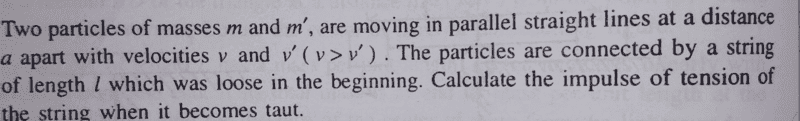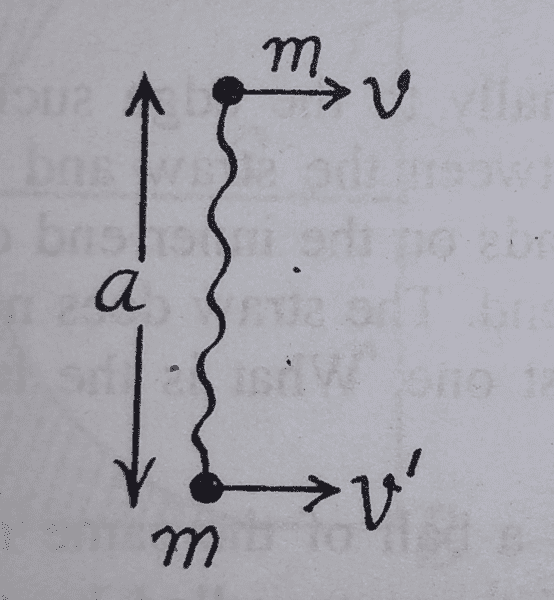# Calculate the impulse of tension

Amitayas Banerjee

## Homework Statement## Homework Equations

$$J \space (\text{Impulse})= \Delta (mv) = F \times \Delta (t)$$

## The Attempt at a Solution

As much I interpreted, we have to calculate the impulse caused by the tension till the relative velocity of approach along the string becomes 0. T to this, I need to figure out how the system moves after the string becomes taut. I have no idea how to do that.

#### Attachments

Cutter Ketch
Well, you’re right, you will need to have an idea of how the system moves after the collision. Try thinking about it in the center of mass coordinates. That should give you an idea.

•Chestermiller
Homework Helper
Gold Member
how the system moves after the string becomes taut.
Think about components of velocity along and normal to the string. What can you about each after it becomes taut? What conservation law can you apply?

Homework Helper
Gold Member
Should I now break the velocities into orthogonal components?
Yes.

What conservation laws do you know for mechanics? Which might apply here?

Amitayas Banerjee
Should I conserve momentum along the string? But how can I? The string prevents a free movement along it, but I can only conserve momentum along an axis which allows free movement along it.

Mentor
Going back to post #2 by @Cutter Ketch . What are the velocity components of the center of mass before the string becomes taut? What are the velocity components of the center of mass after the string becomes taut? At the moment that the string gets taut, where is the center of mass located? What is the movement of each of the masses relative to the center of mass after the string becomes taut?

Cutter Ketch
Should I conserve momentum along the string? But how can I? The string prevents a free movement along it, but I can only conserve momentum along an axis which allows free movement along it.

When to conserve momentum: by Newton’s first law an object will continue in its state of motion unless acted on by a force.

Is m1 acted on by a force external to m1? Yes. Is m2 acted on by a force external to m2? Yes. Is the string acted on by a force outside the string? Yes. BUT as a whole is the system of m1, m2, and the string acted on by a force outside that system? No! So what momentum is conserved? (and don’t forget linear and angular)